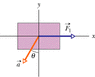# Conceptual question about Newton second law

• edgarpokemon

## Homework Statement

There are two forces on the 2.08 kg box. F1 is 20.5N. acceleration is happening in the third quadrant with a magnitude of 12.1m/s^2 and with an angle of 25.9 (in the third quadrant). Find the second force in vector notation.

Fnet=ma

## The Attempt at a Solution

My question is this. In the picture, the acceleration is occurring at the third quadrant. So I assume that the second force will have negative x and y components, because acceleration has also negative x and y components in that quadrant. So for the x-component of the acceleration, I used F1-F2=m(-acos(25.9)) so F2=m(acos(25.9)+F1. I am assuming acceleration is negative because it is pointing down. in the solution, it tells me that I should use instead F1+F2=ma. But why is there a + sign in F2? since F2 will point in the opposite direction of F1, shouldn't there be a negative sign for F2 instead?

#### Attachments

•fig05_32.gif
2.2 KB · Views: 417